Next: Weak laws of large Up: Probabilistic Encryption Previous: Probabilistic Encryption   Contents

## The Goldwasser-Micali encryption system

As in many previously discussed systems, the person receiving messages chooses two primes (digits)and announces. This system is concerned with whether, for a given number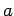, there is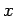with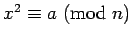. Suchare called squares or (in most books and papers) quadratic residues. For technical reasons, when we refer to squares mod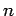, we will excludewhich are divisible by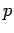or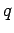. The following facts are easy to prove, in some cases using primitive roots.

Lemma 20   Ifare squares, then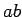is a square. Ifis a square and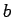is not a square, thenis not a square.

Lemma 21is a square modif and only if it is a square modand a square mod.

Lemma 22   Let. Ifis a square mod,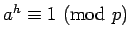. Ifis not a square,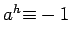.

This implies that, ifandare known, it is easy to decide whetheris a square. The encryption system depends on the assumption (called QRA in the paper [p. 294]) that this problem is very difficult ifare unknown.

Lemma 23of the numbers from 1 toare squares mod. Take the numbers from 1 toand leave out those divisible byor by. Divide the remaining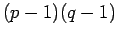numbers into four groups according to whether they are squares or not modand also mod. There arenumbers in each group.

The numbers which are not squares modand also not squares modare called pseudo-squares. Example: If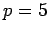,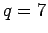, the squares mod 35 are 1, 4, 9, 16, 29, 11 (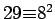,; note we don't include 25 and 14, because they're divisible by). The pseudo-squares must be congruent to 2 or 3 mod 5 and to 3, 5, or 6 mod 7. Thus the pseudo-squares are 17, 12, 27, 3, 33, 13.

The encryption system is primarily concerned with the union of the set of squares and pseudo-squares-- this set is unfortunately denoted both by(p. 291) and by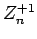. Since exactly half the members ofare squares, the crude idea of saying this is a square'' all the time will only be right half the time. (QRA) says that no algorithm that runs in a reasonable amount of time can do much better than this. [the precise definitions of reasonable'' and much better'' are what require the concepts of circuits of sizeand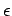-approximating'']

In addition to announcing, the person receiving messages announces one pseudo-square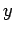. To send a sequence of 0's and 1's, the sender converts them into numbers as follows: for each number in the sequence, anis chosen at random. 0 is converted intomod n, 1 is converted into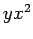. Each 0 or 1 in the sequence can be converted (depending on the choice of) into one ofdifferent numbers. If the message is of length 500 (about one line of ordinary text), and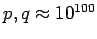, the message can be encoded into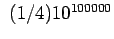different possible ciphertexts.

By Lemma 20, 0's are converted to squares, 1's are converted to pseudo-squares. Since the receiver knows, Lemmas 21 and 22 show he can efficiently decode the message.

In the subsequent sections, we will give the essential ideas of Goldwasser & Micali's proof that (assuming QRA) this system will prevent the adversary from obtaining any partial information about the plaintext.Next: Weak laws of large Up: Probabilistic Encryption Previous: Probabilistic Encryption   Contents
Translated from LaTeX by Scott Sutherland
2002-12-14